```recitation 14: environment diagrams

-----------------------------------------------------
Why bother?

* To formalize the scoping rules we've sorta ignored til now.

* This is how scheme is implemented!  An environment is a list
of frames, a frame is a list of bindings, and a binding is a
list/pair of name & value! (We'll see this later in the term).

-----------------------------------------------------
Just follow the rules, no thinking required!

* If it's a name, start at the current frame and find the closest
binding (walk up the chain of frames as necessary).  If you don't
find it, error!

* If it's a define, create a binding for this variable in the
current frame.  If a binding already exists in the current frame,
replace it.

* If it's a set!, start at the current frame and find the closest
binding of that variable (walk up the chain of frames as necessary).
Change that binding to the new value.  If you don't find a binding,
error!

* If it's a lambda, create a double bubble.  First part points to
the code (params & body).  Second part points to the current
environment (where we are when we create the procedure).

* If it's a procedure application (a combination):

1. Evaluate all the subexpressions.

2. Hang a new frame from the environment pointed to by the
environment pointer of the procedure we are applying.

3. Create bindings in the new frame for the parameters listed in
the code part of the procedure.

4. Evaluate the body of the procedure in the new frame.

-----------------------------------------------------
Notes:

* We start with a global environment that has all the built-in stuff
defined.  It's sometimes handy to draw the built-in's we use.

* As we're evaluating stuff, we always have a current environment
(pointer to one frame that in turn points upwards to other frames,
stopping at the global environment).

* Where to use different colors: Every procedure is a different
color.  When we apply a procedure, draw the new frame in the
same color as the procedure being applied.  (Check: All blue
frames should point to the same place as the environment pointer
of the blue procedure, etc.)

-----------------------------------------------------

(define (fact n)
(if (= n 1)
1
(* n (fact (- n 1)))))
(fact 3)  -->

```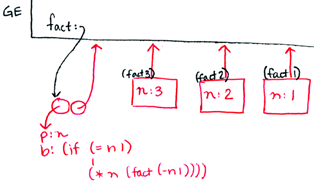```
---------------------------------

(define x 0)
(define f
(lambda (y)
(define x (+ y 10))
x))
(define g
(lambda (y)
(set! x (+ y 10))
x))
(f 5)  -->
x      -->
(g 5)  -->
x      -->

```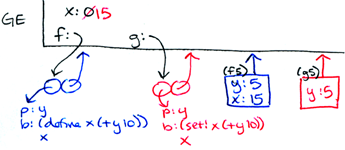```
---------------------------------

(define x 3)
((lambda (x y) (+ (x 1) y))
(lambda (z) (+ x 2))
3)  -->

```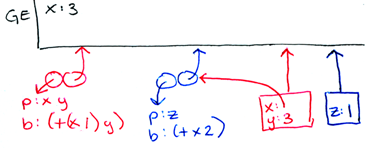```
---------------------------------

(define x 4)
(let ((x (+ 2 1))
(y (square x)))
(* x y))  -->

DESUGARS TO:

((lambda (x y) (* x y))
(+ 2 1)
(square x)) -->

```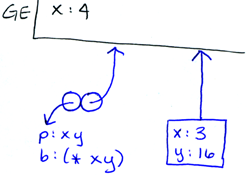```
---------------------------------

(define x 5)
(let ((x (lambda (x) (+ 6 x))))
(set! x (x 7))
x)  -->

```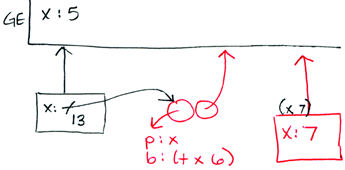```
---------------------------------

(define a 5)
(define foo
(let ((a 10))
(lambda (x)
(+ x a))))
(define (bar a) (foo 20))
(bar 100)  -->

```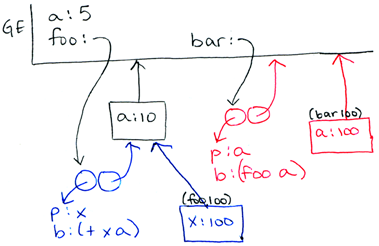```
---------------------------------

(define (make-count-proc f)
(let ((count 0))
(lambda (x)
(if (eq? x 'count)
count
(begin (set! count (+ count 1))
(f x))))))

(define sqrt* (make-count-proc-2 sqrt))
(define square* (make-count-proc-2 square))

(sqrt* 4)  -->
(sqrt* 'count)  -->

(square* 4)  -->
(square* 'count)  -->

```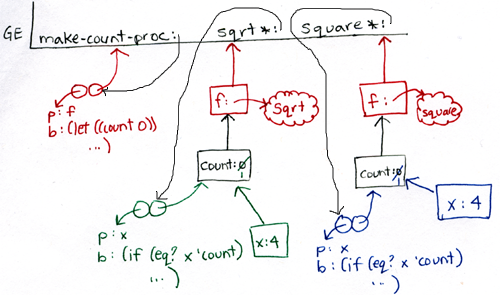```---------------------------------
```